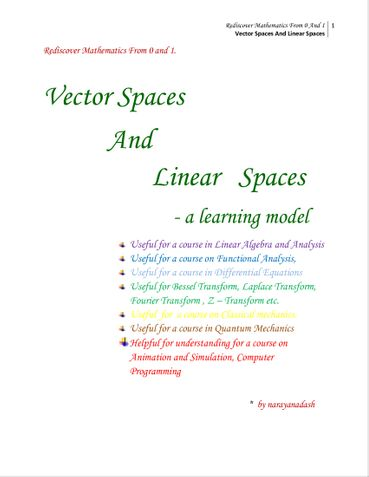You can access the distribution details by navigating to My pre-printed books > Distribution

## Vector Spaces and Linear Spaces (eBook)

Type: e-book
Genre: Mathematics
Language: English
Price: ₹100
(Immediate Access on Full Payment)
Available Formats: PDF

## Description

Vector space is a generalisation of vectors. For example, addition and multiplication of two functions f(x) and g(x) is done point by point , i.e., (f+g)(x) = f(x) +g(x), for each point x in the common domain. Multiplication of a function f(x) by a scalar 'a' is also defined point by point i.e. (af)(x) = af(x) for every point x in the domain of the function. This is like addition of two vectors component by component. What is important is 'linearity' i.e., (af+bg)(x) = af(x) +bg(x). Linearity means 'closure' in other words, i.e., one cannot get out of the set merely by addition or scalar multiplication of elements of the set. Vectors cannot be written without being multiplied by a scalar; e.g., any vector v means 1.v; just like a consonant cannot be pronounced without being combined with a vowel. These are the features that characterise vector spaces, rightly called linear spaces, whether there is a 'magnitude' and 'direction' defined or not. In fact the generalisation of the concepts of magnitude and direction is 'norm' of 'length' of element which is defined in terms of 'inner product' of two elements meaning some sort of ' distance between them' , a generalisation of 'dot product' of two vectors, or elements of a linear space. The 'direction' is specified by 'angles' which is defined in terms of inner product. The 'structure' of these spaces is further revealed by analysing linear transformations or linear mappings between them. The study of linear transformations pave the way for solution of algebraic equations, differential equations, analysis of convergence of series, topology, functional analysis, and quantum mechanics, among a number of other applications.

This is 15th book by the author in the series "Rediscover Mathematics From 0 And 1", who believes that entire mathematics can be rewritten in terms of only three fundamental super concepts, identity, division and symmetry.

## Book Details

Number of Pages: 69

## Ratings & Reviews### Vector Spaces and Linear Spaces

(Not Available)Currently there are no reviews available for this book.

Be the first one to write a review for the book Vector Spaces and Linear Spaces.

## Other Books in Mathematics

#### Shop with confidence

Safe and secured checkout, payments powered by Razorpay. Pay with Credit/Debit Cards, Net Banking, Wallets, UPI or via bank account transfer and Cheque/DD. Payment Option FAQs.